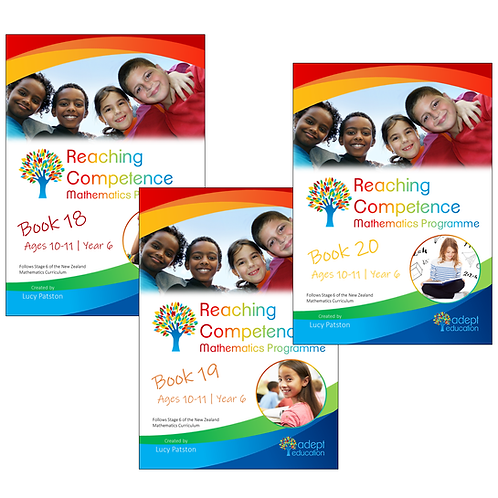`The books cover all of Stage 6 (Years 5 & 6). Book 18 - 94 pages (47 worksheets)Book 19 - 94 pages (47 worksheets)Book 20 - 96 pages (50 worksheets) Get these activity books in PDF format immediately upon purchase. View the sample of Book 19 hereView the sample of Book 20 here`

# Books 18-20 Set e-Copy 2021 Edition

\$25.85Price
• Book 18, Book 19 and Book 20 in PDF form.

View the sample of Book 19 here

View the sample of Book 20 here

Books 18, 19 and 20 cover 27 learning intentions for children aged about 9 to 11 years old.

At this level, students are learning to choose the best way to solve an addition or subtraction problem from a range of strategies. They see numbers as ‘whole’ units but also understand that ‘nested’ within these units is a range of possibilities for partitioning (breaking up) and recombining. This is called part-whole thinking.

For example, 29 + 28 = ?

Using compensation: 30 + 27 or 30 + 28 – 1

Using place value partitioning: 29 + 20 + 8

or 20 + 20 + 9 + 8.

They can also derive multiplication from known facts, e.g., 3 x 8 = 24, so 6 x 8 = 24 + 24 = 48. By now they should know their times tables, decimal numbers to three places and be able to read any fraction.

You will also get: